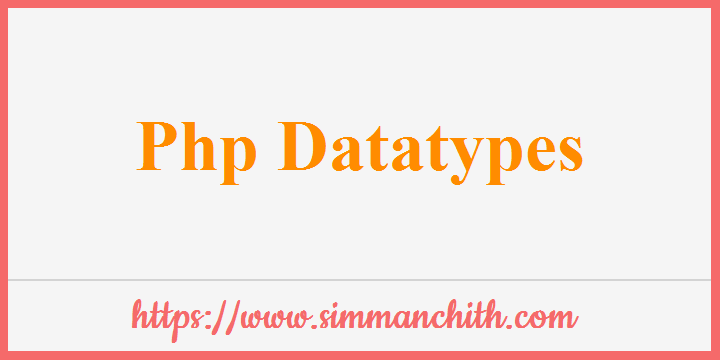# PHP Data TypesVariables can hold several types of data, and different data types can accomplish various functions.

PHP supports the following data types:

• String
• Integer
• Float (floating point numbers - also called double)
• Boolean
• Array
• Object
• NULL
• Resource
You can also search for these topics, data types in php, list the data types in php, what are the data types available in php?, which data types php supports?.

## PHP String

A string is a collection of characters, such as "Hello, world!".

Any text enclosed in quotations can be used as a string. You have the option of using single or double quotations:

Example :-

``````<?php
\$x = "Hello world!";
\$y = 'Hello world!';
echo \$x;
echo "<br />";
echo \$y;
?>
``````

Output :-

Hello world!
Hello world!

You can also search for these topics, define string in php, php string example, php print the string, php string declarations, php string quotes, which type of quotes are used in php?.

## PHP Integer

A non-decimal number between -2,147,483,648 and 2,147,483,647 is considered an integer data type.

Rules for integers

• At least one digit is needed for an integer.
• An integer cannot contain a decimal point.
• The value of an integer might be positive or negative.
• Integer can be defined in decimal (base 10), hexadecimal (base 16), octalÂ (base 8), or binary (base 2).

Example :-

``````<?php
\$x = 5985;
\$y = -26;
?>
``````
You can also search for these topics, integers using php, php integer example, php integer variable, php integer declaration, rules to define integer using php, php integer datatype, php integer range, php integer base.

## PHP Float

A float (a floating-point number) is a decimal or exponentially expressed number.

The float data type can generally hold values up to 1,7976931348623E+308 (depending on platform) with a maximum accuracy of 14 digits.

Example :-

``````<?php
\$x = 10.365;
\$y = 3.1444444444788;
\$z = 1111222222233333.22222222222;
?>
``````
You can also search for these topics, float using php, php float example, php float datatype, php float value, php float function, php float typecast, php float precision.

## PHP Boolean

The two potential states of a Boolean are TRUE and FALSE.

Example :-

``````<?php
\$x = true;
\$y = false;
?>
``````

Booleans are often used in conditional testing.

You can also search for these topics, boolean using php, what are states available in php boolean?, where we can use boolean, php boolean check, php boolean datatype.

## PHP NULL Value

Null is a unique data type that has just one possible value `NULL`.

A value of NULL is automatically allocated if a variable is created without a value.

Note:- NULL means NOTHING. A null is not equal to anything. It cannot compare with anything like text, numbers, and so on.

Example :- Setting the value of a variable to NULL will also empty it:

``````<?php
\$x = "Hello!";
echo \$x . "<br />";
\$x = null;
echo \$x;
?>
``````

Output :-

Hello!
NULL

You can also search for these topics, null value using php, php null or empty, php null example, null check in php, php null datatype, null examples in php.

## PHP var_dump() Method

The `var_dump()` function is used to find the datatype of a given input value.

Example :-

``````<?php
\$a = 10;
\$b = 5.34;
\$c = "Hello";
\$d = true;
var_dump(\$a);
var_dump(\$b);
var_dump(\$c);
var_dump(\$d);
?>
``````

Output :-

int(10)
float(5.34)
string(Hello)
boolean(true)

## PHP Array

An array holds numerous values in a single variable.

Example :- In the example below \$cars is an array. Returns data type and value to PHP var dump():

``````<?php
\$cars = array("Volvo","BMW","Toyota");
var_dump(\$cars);
?>
``````

Output :-

array(3) {
=>
string(5) "Volvo"
=>
string(3) "BMW"
=>
string(6) "Toyota" }

You can also search for these topics, array using php, php array example, php array function, php array types.

## PHP Object

The two basic components of object-oriented programming are classes and objects.

A class is an object template, while an object is a class example.

When creating separate objects (Volvo, BMW, Toyota, etc.) all of the attributes and behaviors of the class belong to them, but each object will have distinct property values.

Let's pretend we have a Car class. A car can have characteristics such as model, color, and so on. To hold the values of these properties, we can define variables like `\$model`, `\$color`, and so on.

When you create an object from a class, PHP will call you to the __construct() method.

Example :-

``````<?php
class Car {
public \$color;
public \$model;
public function __construct(\$color, \$model) {
\$this->color = \$color;
\$this->model = \$model;
}
public function message() {
return "My car is a " . \$this->color . " " . \$this->model . "!";
}
}
\$myCar = new Car("black", "Volvo");
echo \$myCar -> message();
echo "<br />";
\$myCar = new Car("red", "Toyota");
echo \$myCar -> message();
?>
``````

Output :-

My car is a black Volvo!
My car is a red Toyota!

You can also search for these topics, object in php, object methods in php, php object example, how to create an object?, php object declaration, what is an object?, object creation in php.

## PHP Resource

The particular type of resource is a real form of data. It stores a reference to external PHP functions and resources.

A database call is a popular example of how to use the resource data type.

You can also search for these topics, Resource datatype, resource using php, php resource id, php resource index.